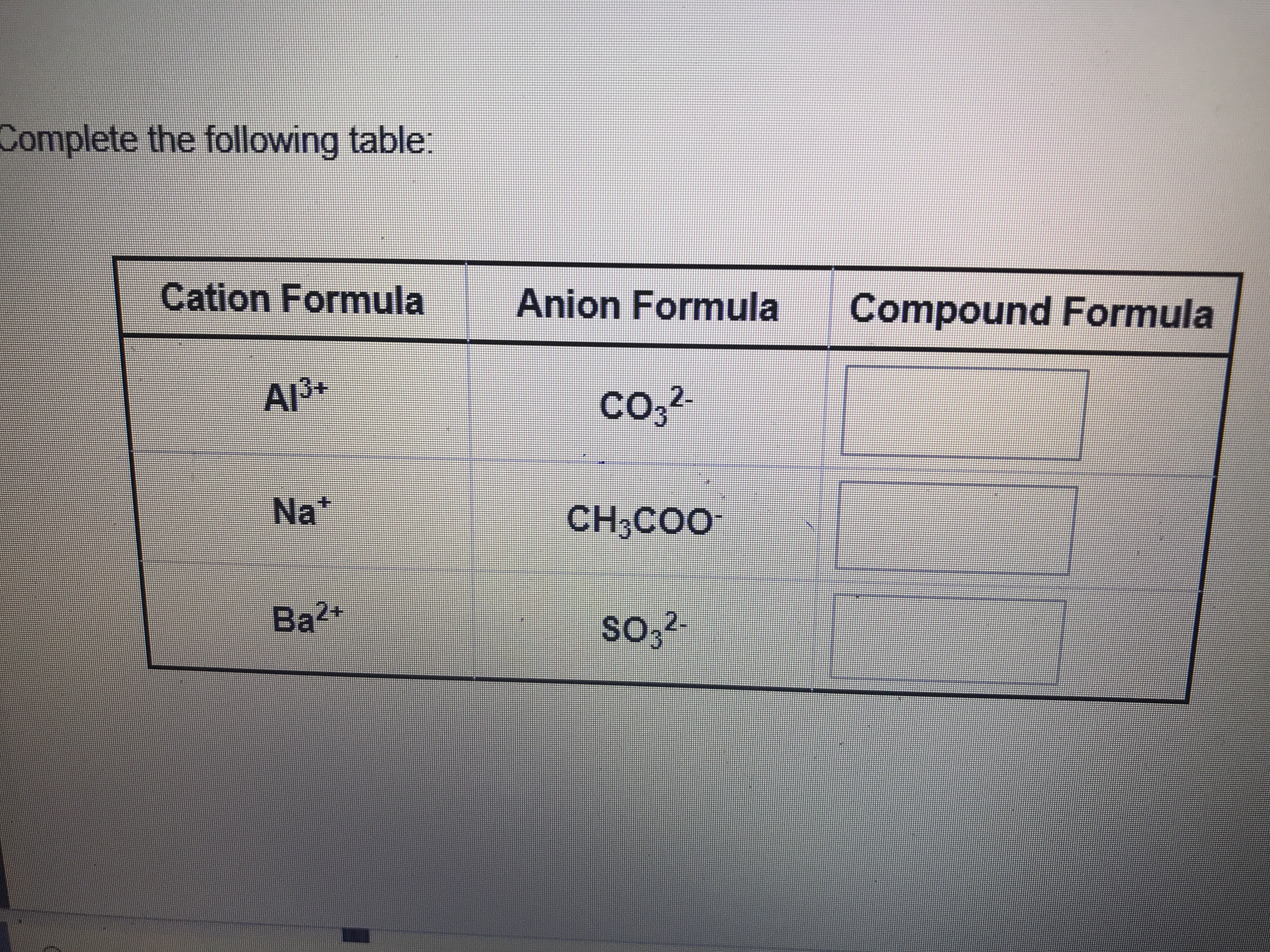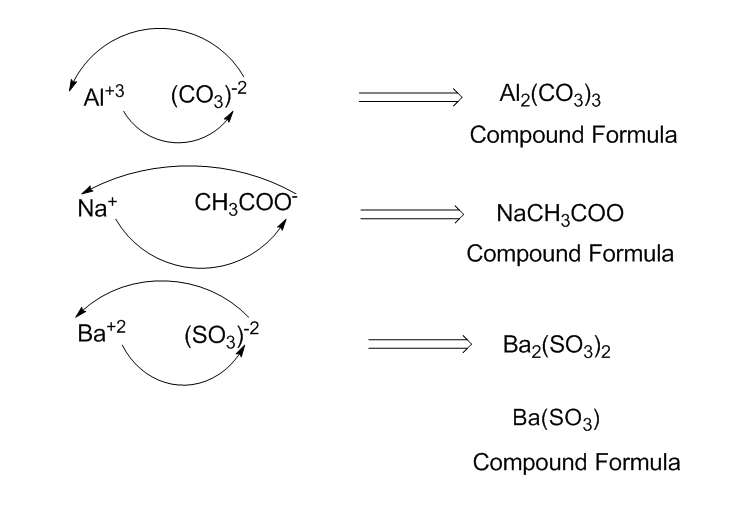# Complete the following tableCation FormulaAnion FormulaCompound FormulaА)З-co,2-Na*CH;COOBaso,2-

Question
2 viewshelp_outlineImage TranscriptioncloseComplete the following table Cation Formula Anion Formula Compound Formula А)З- co,2- Na* CH;COO Ba so,2- fullscreen
check_circle

Step 1

The superscript present in ions represent the charges. Always the final chemical molecule has to be in neutral form.

Therefore, the simplest way to determine the compound formula is as follows,

First the chemical symbol has to be written.

Then charges have to be considered.

Crossing the charge will give us the compound formula.

Finally, if possible lower the numbers from the obtained formula so that the lowest whole number is achieved.

Step 2...

### Want to see the full answer?

See Solution

#### Want to see this answer and more?

Solutions are written by subject experts who are available 24/7. Questions are typically answered within 1 hour.*

See Solution
*Response times may vary by subject and question.
Tagged in

### Chemistry